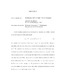## Families of Cyclic Cubic FieldsShanks considered what he termed the simplest cubic fields,'' defined as the splitting fields of the polynomials \begin{equation} S_n = X^3+(n+3)X^2+nX-1. \end{equation} In particular, he showed that if the square root of the polynomial discriminant is squarefree, then the roots of $S_n$ form a system of fundamental units for its splitting field. The analysis of this family was extended by Lettl and Washington. Lecacheux, and later Washington, discovered a second one-parameter family with a similar property: if a certain specified chunk of the polynomial discriminant is squarefree, the roots of the polynomial form a system of fundamental units. Kishi found a third such family. In the following, we show that there are many, many more families of cubics with this property. We generalize the model of Washington, explicitly exhibit new families of cyclic cubic fields, and interpret all known and new families as curves on the elliptic surface $X(3)$.